# Fur Rendering

#### Notes:

This tutorial was originally hosted on www.sgtconker.com. Sgt. Conker is no longer online, so here is the original article, reprinted. Enjoy!

# Fur Rendering in XNA

In this article, you will see how you can render fur in your XNA games. At first, we will look over the technique use to render the fur, then we will look at several improvements that can be done, and in the end, we will apply it to a model.

## Rendering Fur

When thinking about rendering fur, there are a few possible ways you could think of. The most obvious is to model each individual strand of hair by one or two polygons. This way, you could animate each strand individually, but the cost of modeling and rendering just a small patch of hair would be awfully large. Another way would be to make some polygons which are rendered with textures containing some strand of hair on them, and transparency between them (one polygon contains a vertical slice of a hair patch). This is also fairly easy to animate, but it yields some problems when trying to use alpha blending (because you need to sort the polygons) and it looses it’s furriness when viewed from above. The method that will be implemented in this sample is called shell rendering, and creates a patch of fur by rendering several horizontal slices through the patch. As opposed to the previous method, the problem with this approach is when viewing the patch of hair from low angles (looking at it from the side). However, when applying this to a model, instead of a flat surface, this artifact is not so visible, and we can get away with it. Another possibility would be to combine the shell rendering with the vertical slices, and obtain a method called shells and fins. But this will be left as future improvements. For the scope of this tutorial, we will only focus on the shells.

#### Rendering with shells

The main idea of rendering with shells can be illustrated through the following image.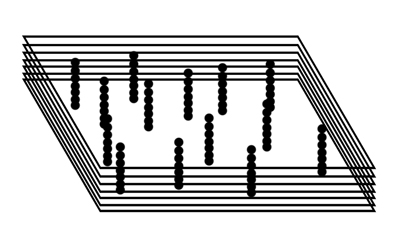As you can see, there are several horizontal slices through the patch of hair. Each slice by itself doesn’t to a good job of representing fur: it is just a polygon with lots of points on it. But when stacking enough slices one over the other, the impression of fur soon emerges. It’s just like drawing a line by drawing lots of points close to each other. To render fur like this, there are three main issues that have to be considered:

• Information about the positions of the hair strands. When rendering each slice, we need to know which pixels will be visible (belonging to a strand of hair), and which pixels will be transparent. We will store this information in a texture. A opaque pixel in this texture will represent a hair strand. When creating this texture, we will randomly place opaque pixels on it. The number of pixels we will place will depend on a value which will indicate the density of the hair.
• Position of the slices. For a simple demonstration on a planar horizontal patch, the positions of the slices would simply be growing on the Up axis. However, we want to make this easily applicable on any model, so the best way to do this is to displace each slice based on the base polygon’s normal. In fact, to create the slices, we will just render each polygon several times, and at each rendering, we will displace the polygons with a small distance, based on their normals.
• Color of each hair strand. Initially, we will simply read the color from the density map, but afterwards, we will read the color from a separate map, in order to achieve nicer looking fur.

Next, we will go through rendering a patch of fur, step by step, starting with generating the fur texture.

#### Generation of the fur map

As seen previously, the fur map will be used to render each slice of fur. It will contains transparent pixels for non-fur areas, and opaque pixels for hair strands. We compute the number of these pixels using the density, and then randomly place them on the surface of the texture. The complete function that does this can be seen below.

```///
<summary> /// This functions prepares a texture to be used for fur rendering
/// </summary>
///This will contain the final texture
///Hair density in [0..1] range
private void FillFurTexture(Texture2D furTexture, float density)
{
//read the width and height of the texture
int width = furTexture.Width;
int height = furTexture.Height;
int totalPixels = width * height;

//an array to hold our pixels
Color[] colors;
colors = new Color[totalPixels];

//random number generator
Random rand = new Random();

//initialize all pixels to transparent black
for (int i = 0; i < totalPixels; i++)
colors[i] = Color.TransparentBlack;

//compute the number of opaque pixels = nr of hair strands
int nrStrands = (int)(density * totalPixels);

//fill texture with opaque pixels
for (int i = 0; i < nrStrands; i++)
{
int x, y;
//random position on the texture
x = rand.Next(height);
y = rand.Next(width);
//put color (which has an alpha value of 255, i.e. opaque)
colors[x * width + y] = Color.Gold;
}

//set the pixels on the texture.
furTexture.SetData(colors);
}
```

After having the fur texture, we will first try to render fur on a single polygon. For simplicity, we will simply use an array of vertices, which will be drawn using DrawUserPrimitives. Later in the tutorial, we will use the Model class.

#### Generating the geometry

The function that generates geometry simply creates two triangles, sitting on the XY plane, with the -Z axis as a normal, and corresponding texture coordinates.

```VertexPositionNormalTexture[] vertices;

private void GenerateGeometry()
{
vertices = new VertexPositionNormalTexture;
vertices = new VertexPositionNormalTexture( new Vector3(-10,0,0),
-Vector3.UnitZ,
new Vector2(0,0));
vertices = new VertexPositionNormalTexture( new Vector3(10,20,0),
-Vector3.UnitZ,
new Vector2(1,1));
vertices = new VertexPositionNormalTexture( new Vector3(-10, 20, 0),
-Vector3.UnitZ,
new Vector2(0, 1));

vertices = vertices;
vertices = new VertexPositionNormalTexture( new Vector3(10, 0, 0),
-Vector3.UnitZ,
new Vector2(1, 0));
vertices = vertices;
}
```

#### Rendering the shells

We saw earlier the we need to draw a certain number of slices in order to achieve the wanted effect. The slices have to each be displaced by a small amount from the previous slice. Generating all these displacements on the CPU is a time consuming work, and since we have access to vertex shaders, it would be a pity to waste all those CPU cycles for something the GPU can do easily. To achieve all layers, we will send the same geometry several times to be rendered. We will also tell the shader which slice we are currently rendering, and what is the maximum displacement from the base geometry. Using this information, the vertex shader will slightly modify the position of each vertex along the normal, based on the current layer.

Now that you have the fur texture, and the geometry, the next step is to write the shader that will handle the rendering of fur. To do this, create a new shader in the Content project. For the shader, we will need several parameters. The World, View and Projection matrices are well-known, but we also need a parameter that controls the hair length, let’s name it MaxHairLength, and one that tells us which horizontal slice (i.e. layer) is currently being processed. To achieve some independence from the actual numbers of layers that are actually used, we will consider that the CurrentLayer parameter belong to the [0..1] range, 0 meaning that the current layer is the closest to the real surface, and 1 that it is the farthest from it. The intermediate layers will map to intermediate values. This value, multiplied with the maximum hair length will yield the displacement of the layer being processed at the respective moment. Lastly, we need to read data from the fur texture, so we need a parameter and a sampler for it.

```float4x4 World;
float4x4 View;
float4x4 Projection;

float CurrentLayer; //value between 0 and 1
float MaxHairLength; //maximum hair length

texture FurTexture;
sampler FurSampler = sampler_state
{
Texture = (FurTexture);
MinFilter = Point;
MagFilter = Point;
MipFilter = Point;
};
```

Each vertex needs to have data related to position, normal and texture coordinates. This data is send by the application, so we need to declare a vertex input structure that can receive it. The output of the vertex shader will contain the final position, and the texture coordinates. Later in the tutorial, when you will also add lighting to the fur, the normals will also be passed as an output, but for now, we leave that out. The two vertex shader structures can be seen below.

```struct VertexShaderInput
{
float3 Position : POSITION0;
float3 Normal : NORMAL0;
float2 TexCoord : TEXCOORD0;
};

{
float4 Position : POSITION0;
float2 TexCoord : TEXCOORD0;
};
```

Now, to move onwards to the main part of the effect file, the shaders. In the vertex shader, you need to generate the new position of a vertex, as explained previously. To obtain the new position, we compute the current layer’s displacement, and move the vertex along its normal with that value. The line of code that does that is:

```float3 pos = input.Position + input.Normal * MaxHairLength * CurrentLayer;
```

This results in the final position of the vertex. The rest of the vertex shader implements the operations commonly found in vertex shaders: transform the position using the three matrices, and pass along the texture coordinates to the pixel shader. The final vertex shader can be seen below.

```VertexShaderOutput FurVertexShader(VertexShaderInput input)
{
float3 pos;
pos = input.Position + input.Normal * MaxHairLength * CurrentLayer;

float4 worldPosition = mul(float4(pos,1), World);
float4 viewPosition = mul(worldPosition, View);
output.Position = mul(viewPosition, Projection);

output.TexCoord = input.TexCoord;
return output;
}
```

The pixel shader simply reads from the fur texture, and output the color. Since we convened that the fur shader has alpha 0 for areas where there is no hair, we will use alpha blending to make those areas transparent. The pixels which belong to the hair strands will remain opaque. Specifying the alpha blending can be done either through code in the application, or inside the declaration of techniques and passes in the effect files. For this tutorial, we chose the latter.

```float4 FurPixelShader(VertexShaderOutput input) : COLOR0
{
return tex2D(FurSampler, input.TexCoord);
}

technique Fur
{
pass Pass1
{
AlphaBlendEnable = true;
SrcBlend = SRCALPHA;
DestBlend = INVSRCALPHA;
CullMode = None;

}
}
```

#### Putting it together: drawing the geometry

For clearer code, let’s create a function that draws the geometry we defined earlier. This function needs to set the vertex declaration on the device, and make a call to GraphicsDevice.DrawUserPrimitives.

```private void DrawGeometry()
{
using (VertexDeclaration vdecl = new VertexDeclaration(
GraphicsDevice,
VertexPositionNormalTexture.VertexElements))
{
GraphicsDevice.VertexDeclaration = vdecl;
GraphicsDevice.DrawUserPrimitives(PrimitiveType.TriangleList, vertices, 0, 2);
}
}
```

Now we need to write some code in the Draw function, code that actually draws our object and the fur layers. Before this, we need to add some members, and initialize them in the LoadContent() function. Also add the file Camera.cs to your project, and initialize a camera in the game’s Initialize function.

```[...]
using XNASimpleCamera; //namespace of Camera.cs
[...]

public class Game1 : Microsoft.Xna.Framework.Game
{
[...]

//simple camera for use in the game
Camera camera;
//texture containing fur data
Texture2D furTexture;
Effect furEffect;
//number of layers of fur
int nrOfLayers = 40;
//total length of the hair
float maxHairLength = 2.0f;
//density of hair
float density = 0.2f;

protected override void Initialize()
{
//initialize the camera
camera = new Camera(this);
base.Initialize();
}

{
[...]
//generate the geometry
GenerateGeometry();
//create the texture
furTexture = new Texture2D(GraphicsDevice,
256, 256, 1,
TextureUsage.None,
SurfaceFormat.Color);
//fill the texture
FillFurTexture(furTexture, density);
}
}
```

You can use some of the parameters to change the way the fur looks and behaves. The numberOfLayers controls both the quality and the performance. A large number of layers will result in better quality, but may result in some loss of performance. You can easily reach a compromise depending on your application. The maxHairLength and density easily modify the looks of the fur.

Now let’s go to the Draw() function. Here we need to set up the parameters for the effect. After that, we draw the mode nrOfLayers times, and set the CurrentLayer shader parameter before each drawing.

```protected override void Draw(GameTime gameTime)
{
graphics.GraphicsDevice.Clear(Color.CornflowerBlue);

furEffect.Parameters["World"].SetValue(Matrix.CreateTranslation(0,-10,0));
furEffect.Parameters["View"].SetValue(camera.View);
furEffect.Parameters["Projection"].SetValue(camera.Projection);
furEffect.Parameters["MaxHairLength"].SetValue(maxHairLength);
furEffect.Parameters["FurTexture"].SetValue(furTexture);

furEffect.Begin();
for (int i = 0; i < nrOfLayers; i++)
{
furEffect.Parameters["CurrentLayer"].SetValue((float)i/nrOfLayers);
furEffect.CommitChanges();
furEffect.CurrentTechnique.Passes.Begin();
DrawGeometry();
furEffect.CurrentTechnique.Passes.End();
}
furEffect.End();
}
```

Running the game after this should show something similar to the following picture.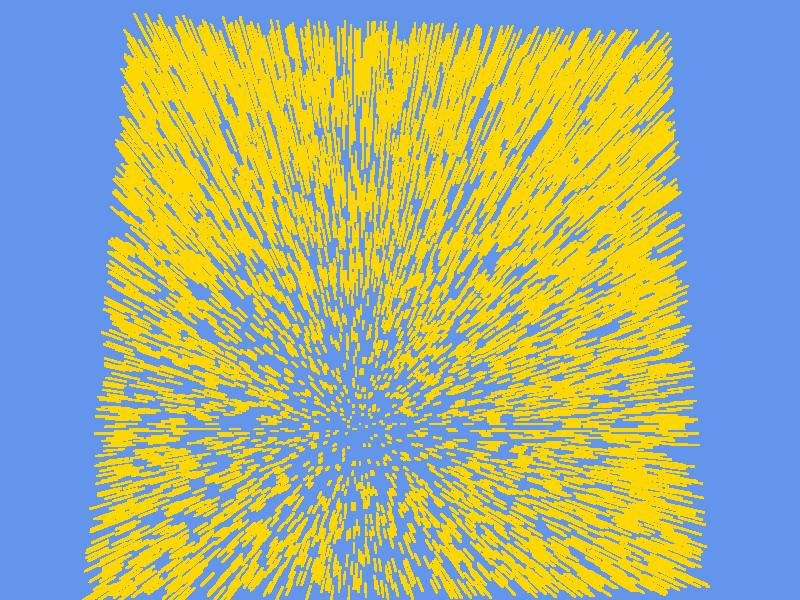As you can see, the strands of fur show up. However, there are two things that could be done better. The first one is drawing an opaque polygon at the base of the fur, so we don’t see through to the other side, and the second is applying colors from a texture to the fur.Now add a member to the Game class to hold this texture, and load it inside the LoadContent() function.

```Texture2D furColorTexture;
{
[...]
}
```

Next, we need to modify the shader. We will add a new parameter and sampler for the color texture. Inside the pixel shader (the only place we need to make modifications), we will read the color information and combine it with the alpha of the fur texture. (Note: we could also just put the transparency information in the color texture, but separating them let’s us easily change the color texture, and leaves us with potential space for parameters that might be written into the RGB channels of the fur texture. We will talk about this later)

In order to make the base layer opaque, we will compare the CurrentLayer variable with 0. If it is 0, we set the result’s alpha to 1 (opaque), otherwise, we set it to the value read from the fur texture.

```texture Texture;
sampler FurColorSampler = sampler_state
{
Texture = (Texture);
MinFilter = Linear;
MagFilter = Linear;
MipFilter = Linear;
};
[...]
{
float4 furData = tex2D(FurSampler, input.TexCoord);
float4 furColor = tex2D(FurColorSampler, input.TexCoord);

//make opaque if lowest layer, otherwise use alpha channel of furData
furColor.a = (CurrentLayer == 0) ? 1 : furData.a;
return furColor;
}
```

```furEffect.Parameters["Texture"].SetValue(furColorTexture);
```

The result can be seen below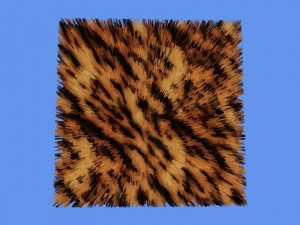## Improvements

#### Improvement #1: Fake Inter-Fur Occlusion

One issue that appears is visible if we were to render the whole fur with the same color. The result (seen below) does not make individual hair strands stand out, and is not visually pleasing.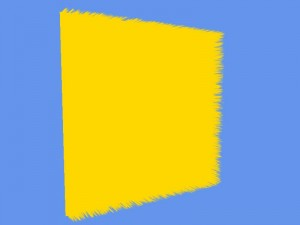We can easily fix this using some fake shadows. In a patch of fur/hair, the lower part of the hair will usually have much lesser light reaching it that the upper layers. This is why we can use the information about the layer currently being drawn (obtainable through the CurrentLayer parameter) to add some fake shadows. The code that handles this should go in the pixel shader of the fur effect.

```    //based on layer depth, choose the amount of shading.
//we lerp between two values to avoid having the base of the fur pure black.
```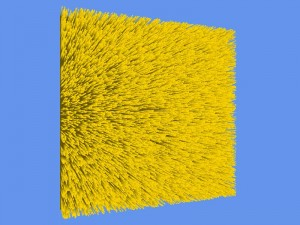As you can see, the hair stands out and much better, and the overall effect is nicer. The look of textured fur is similary improved.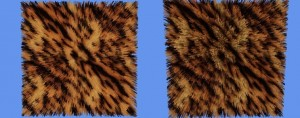#### Improvement #2: Height Variation

We talked earlier about using the RGB channels of the fur texture to add some parameters for more special rendering. We then mentioned rendering fur strands of different height. This is what we will do now.

First, we need to modify the FillFurTexture() function. We will assign to each strand of hair a number which will represent the maximum layer on which that particular strand will be visible. For our example, we will choose to divide the strands by the number of layers. So assuming we have 1000 strands and 10 layers, the first 100 strands (1000/10) will only be one layer high, the second 100 strands wil go up to the second layer, and so un, until the last hundred strands will reach the top. This gives a linear distribution of heights. Let’s see how this looks in the code. We will first compute how many strands reach each layer. Then, when computing the position of each strand, we check to see which group it belongs to, i.e. which is the maximum layer reachable by that strand. Then we normalize this value by dividing it to the total number of layers, to get in into the [0..1] range. Then we set this as a value to the red channel of the pixel.

```private void FillFurTexture(Texture2D furTexture, float density)
{
[...] //other computations
//compute the number of opaque pixels = nr of hair strands
int nrStrands = (int)(density * totalPixels);

//compute the number of strands that stop at each layer
int strandsPerLayer = nrStrands / nrOfLayers;

//fill texture with opaque pixels
for (int i = 0; i < nrStrands; i++)
{
int x, y;
//random position on the texture
x = rand.Next(height);
y = rand.Next(width);

//compute max layer
int max_layer = i / strandsPerLayer;
//normalize into [0..1] range
float max_layer_n = (float)max_layer / (float)nrOfLayers;

//put color (which has an alpha value of 255, i.e. opaque)
//max_layer_n needs to be multiplied by 255 to achieve a color in [0..255] range
colors[x * width + y] = new Color((byte)(max_layer_n * 255), 0, 0, 255);
}
//set the pixels on the texture.
furTexture.SetData(colors);
}
```

To use this data, we need to also modify the pixel shader of the fur effect. Before drawing a pixel, we verify if the maximum layer stored in the red channel of the pixel is lower that the current layer. If it is, we don’t draw the current pixel. If it isn’t (we haven’t reached the maximum layer of this pixel yet), we draw it. We also need to combine this with the test we wrote previously about making the lower layer fully opaque. The two lines that achieve this can be seen below.

```float furVisibility =(CurrentLayer > furData.r) ? 0 : furData.a;
furColor.a = (CurrentLayer == 0) ? 1 : furVisibility;
```

You can compare the results below. The first image uses fixed height for all strands, the second one has linear variations.

One more improvement that can be done related to the height of the hairs stands is to make the height distribution non linear. To do this, just add a line that modifies the value of max_layer_n, right after first computing it. There are several ways to modify this, yielding different results.

For some interesting effects, you can also use the X and Y position to compute the height.

```max_layer_n = 0.2f + 0.8f * ((float)Math.Sin((double)x / height * 20) / 2.0f + 0.5f);
```
```Vector2 dist = new Vector2((float)x / height - 0.5f, (float)y / width - 0.5f);
max_layer_n = 0.4f + 0.6f *( (float)Math.Cos(dist.Length() * 50) / 2.0f + 0.5f);
```You can experiment with different ways of modifying the value of max_layer_n and see what comes up.

#### Improvement #3: Deformation

To add one lat bit of realism to the fur simulation, we can make it move. Since our strands are composed of a number of layers, we can’t move each strand individually, but we can move them all at once. To realize this movement, it is enough to slightly displace each layer (with lower values for lower layers, and larger values for higher layers) in order to give the appearance of deformation. If we alter the displacement value each frame, the fur will look like it’s moving. For a quick exemplification, we will take into consideration the gravity, and some arbitrary force. Let’s declare the needed variables as members of type Vector3. The gravity will point downwards, for now the force vector will be zero, and we also need a final displacement vector. These are declared in the Game class.

```//movement vectors
Vector3 gravity = new Vector3(0, -1.0f, 0);
Vector3 forceDirection = Vector3.Zero;
//final displacement for hair
Vector3 displacement;
```

We also need a parameter for displacement in the shader. Go ahead and at it to the top of the effect file.

```float3 Displacement;
```

Inside the vertex shader this time, we will use this parameter to alter the position of the fur vertices in world space. We don’t want to apply the same displacement for all layers, because the whole fur would move. We will apply multiply the displacement with a factor ranging from 0 to 1, based on the layer being processed. If we would just consider that factor to be CurrentLayer, the displacement would look unrealistic. In real life, a strand of hair is curbed when blown by the wind or affected by gravity, because different parts of the strand have different elasticity and string tension. To simulate this, we will use the third power of the CurrentLayer, to obtain a non-linear displacement. By raising it to a power, the way the displacement grown seems more natural and gives better results. The code can be seen below.

```VertexShaderOutput FurVertexShader(VertexShaderInput input)
{
float3 pos;
pos = input.Position + input.Normal * MaxHairLength * CurrentLayer;

float4 worldPosition = mul(float4(pos,1), World);

//make the displacement non linear, to make it look more like fur
float displacementFactor = pow(CurrentLayer, 3);
//apply the displacement
worldPosition.xyz +=Displacement*displacementFactor ;

return output;
}
```

Back to the drawing code, add the following two lines before anything else in the Draw() function. They compute the final value of the displacement, by summing the gravity and the other force (which for now remains zero). After that, the value is set on the effect parameter with the same name.

```displacement = gravity + forceDirection;
furEffect.Parameters["Displacement"].SetValue(displacement);
```

The result is hair affected by gravity.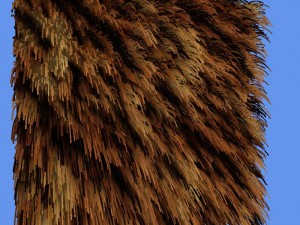Now add a new line at the top of the Draw() function, to modify the forceDirection based on time, and look at the animating fur.

```forceDirection.X = (float)Math.Sin(gameTime.TotalGameTime.TotalSeconds) * 0.5f;
```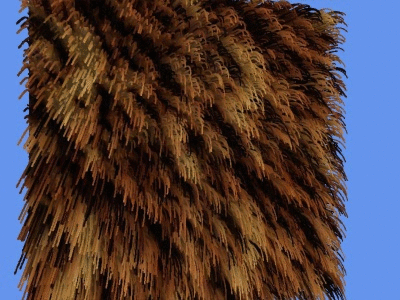You can experiment more by modifying the forceDirection or gravity vectors, or my applying some rotations to the model (furEffect.Parameters[“World”]) and see how the fur behaves.

The complete code for the sample (including textures, fake inter-fur shadows, height variations and animations) can be downloaded here.

## Applying Fur on a Model

We will apply the fur shader on a 3D model, and use the model’s own textures for coloring the fur. Note: the methods used from now on are not the recommended ones. The best approach would be to crate a new Content Processor, and maybe even a custom Model class, and use those on the model that you wish to have fur. However, these operations are beyond the scope of this tutorial.

Add the model of the dinosaur to your Content folder, and make sure the associated textures (dino.png and eyes.png) are inside the same folder as the model. The files can be found in this archive: dino.zip.

Now we need to add a variable to hold the model, and initialize it inside LoadContent().

```//dino model
Model dino;

{
[...]
}
```

We will need two functions. One of them simply draws the geometry of the model. It takes as parameters the model that should be drawn, the collection of bones that will be used, and the effect that will be on the device. It sets the values of the World matrix on the effect, and that of the Texture parameter, so the texture used to color the model’s fur will be the same as the texture set on the model.

```private void DrawModelGeometry(Model model, Matrix[] bones, Effect effect)
{
foreach (ModelMesh mesh in model.Meshes)
{
effect.Parameters["World"].SetValue(bones[mesh.ParentBone.Index]); //set World Matrix
foreach (ModelMeshPart meshpart in mesh.MeshParts)
{
effect.Parameters["Texture"].SetValue(((BasicEffect)meshpart.Effect).Texture); //set Texture
effect.CommitChanges(); //commit changes
graphics.GraphicsDevice.VertexDeclaration = meshpart.VertexDeclaration;
graphics.GraphicsDevice.Vertices.SetSource(mesh.VertexBuffer, meshpart.StreamOffset, meshpart.VertexStride);
graphics.GraphicsDevice.Indices = mesh.IndexBuffer;
//draw the geometry
graphics.GraphicsDevice.DrawIndexedPrimitives(PrimitiveType.TriangleList, meshpart.BaseVertex, 0,
meshpart.NumVertices, meshpart.StartIndex, meshpart.PrimitiveCount);
}
}
}
```

The seconds function applied the fur rendering algorithm on the model, using code similar to what we used previously to apply fur to a single polygon.

```private void DrawFurModel(Model model)
{
Matrix[] bones = new Matrix[model.Bones.Count];
model.CopyAbsoluteBoneTransformsTo(bones);

furEffect.Parameters["Displacement"].SetValue(displacement);
furEffect.Parameters["MaxHairLength"].SetValue(maxHairLength);
furEffect.Parameters["FurTexture"].SetValue(furTexture);

furEffect.Parameters["View"].SetValue(camera.View);
furEffect.Parameters["Projection"].SetValue(camera.Projection);
furEffect.Parameters["MaxHairLength"].SetValue(maxHairLength);
furEffect.Parameters["FurTexture"].SetValue(furTexture);

furEffect.Begin();
for (int i = 0; i < nrOfLayers; i++)
{
furEffect.Parameters["CurrentLayer"].SetValue((float)i / nrOfLayers);
furEffect.CommitChanges();
furEffect.CurrentTechnique.Passes.Begin();
//draw geometry of current layer
DrawModelGeometry(model, bones, furEffect);
furEffect.CurrentTechnique.Passes.End();
}
furEffect.End();
}
```

After tweaking some values, like the dimension of the fur texture and the force and gravity vectors, a simple call to DrawFurModel(dino); inside the Draw() function will result in the image seen below.By adding simple N*L lighting in the fur shader (illustrated in the accompanying source code), the quality of the scene is further improved.The source code for the fur rendering applied to a model can be found here.

## Conclusions

This tutorial showed how to render fur, and apply several effects on it, and finally apply it on a model. I hope you find it useful and entertaining.

The final source code archives are:

• http://gamedev.michaeljameswilliams.com/ MichaelJW

Sadly, Sgt. Conker seems to be offline. Any chance you have a backup of this tut? The screenshots look great!

• Catalin Zima

Hi Michal.

As you can see above, I posted the whole article here.
Code is still with an old version of XNA, but following the tutorial should allow you to reach a functional 4.0 version 🙂

• fuzzball_b

Changing the draw code to the code below made it work in XNA 4.0. the XNA 3.1 to 4 conversion cheatsheet was a big help.

http://nelxon.com/resources/xna-3-1-to-xna-4-0-cheatsheet.php

DepthStencilState ds = GraphicsDevice.DepthStencilState;
BlendState bs = GraphicsDevice.BlendState;

GraphicsDevice.RasterizerState = RasterizerState.CullClockwise;
GraphicsDevice.DepthStencilState = DepthStencilState.Default;
GraphicsDevice.BlendState = BlendState.NonPremultiplied;

for (int shell = 0; shell < 16; shell++)
{
effect.Parameters["ShellOffset"].SetValue(shell / 16f);
effect.CurrentTechnique.Passes.Apply();

foreach (EffectPass pass in effect.CurrentTechnique.Passes)
{
pass.Apply();

foreach (ModelMesh mesh in sharky.Meshes)
{
foreach (ModelMeshPart meshPart in mesh.MeshParts)
{
effect.Parameters["World"].SetValue(GetAbsoluteTransform(mesh.ParentBone) * world);

GraphicsDevice.SetVertexBuffer(meshPart.VertexBuffer);
GraphicsDevice.Indices = meshPart.IndexBuffer;
GraphicsDevice.DrawIndexedPrimitives(PrimitiveType.TriangleList, 0, 0,
meshPart.NumVertices, meshPart.StartIndex, meshPart.PrimitiveCount);
}
}
}
}

GraphicsDevice.DepthStencilState = ds;
GraphicsDevice.BlendState = bs;

• http://ii.ca/13bsx Ii.Ca

We’re a group of volunteers and opening a new scheme in our community.
Your web site provided us with valuable information to work on.

You’ve done an impressive job and our whole community will be thankful to you.